### Home > A2C > Chapter 12 > Lesson 12.3.1 > Problem12-133

12-133.
1. Find the exact value, if possible, for each of the following trig expressions: Homework Help ✎

1. tan(0)

2.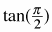3. tan(π)

4.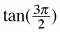5. tan(2π)

6. tan(4π)

7. Explain each of your answers as it relates the graph of y = tan θ.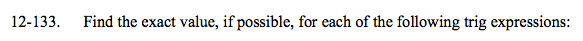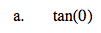0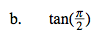undefined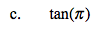Where is this angle on the unit circle?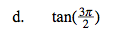Where is this angle on the unit circle?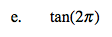Where is this angle on the unit circle?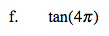Where is this angle on the unit circle?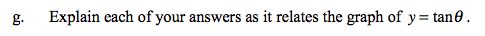What special points do all of these values correspond to?